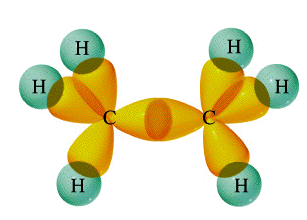Which of the following molecules has five σ bonds and one π bond?

(a) ${\text{C"_2"H}}_{6}$ (b) $\text{C"_2"H"_3"Cl}$ (c) $\text{C"_2"H"_2"Cl}$ (d) ${\text{C"_2"H}}_{4}$

Apr 18, 2017

The correct answer is d) ${\text{C"_2"H}}_{4}$.

Explanation:

a) ${\text{C"_2"H}}_{6}$Ethane has six σ bonds formed by the overlap of ${\boldsymbol{\text{sp}}}^{3}$ and $\text{s}$ orbitals.

b) $\text{C"_2"H"_3"Cl}$This compound has only three σ bonds (the $\text{C-H}$ bonds) formed by the overlap of ${\text{sp}}^{2}$ and $\text{s}$ orbitals.

c) $\text{C"_2"H"_2"Cl}$

This compound does not exist.

d) ${\text{C"_2"H}}_{4}$Ethylene has (i) four σ bonds formed by the overlapping of ${\text{sp}}^{2}$ and $\text{s}$ orbitals;
(ii) one σ bond formed by overlapping of two ${\text{sp}}^{2}$ orbital; and (iii) one π bond formed by overlap of two $\text{p}$ orbitals.